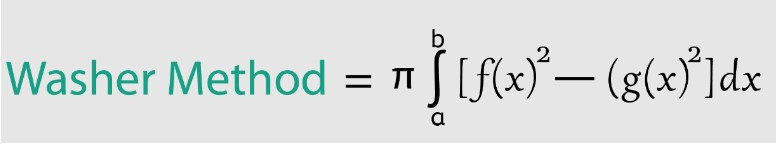# Washer Method Calculator

To calculate volume of the solid revolution, enter f(x), g(x), and upper & lower limit into the required input fields and click t he calculate button using washer calculator

This will be calculated:

Give Us Feedback

## Washer Method Calculator

Washer method calculator finds the volume of the solid revolution to cover the sold with a hole by using a definite integral. This washer calculator finds the definite integral of the sum of two squared functions (f(x)2 + g(x)2) and multiplies it by π (pi).

## What is the washer method?

In geometry, a washer method is used to find the volume of different kinds of solid shapes such as a round shape with a hole in the center. The shapes are obtained by rotating two functions around the x-ais and y-axis.

## Formula of washer method

The formula for the washer method is:## How to calculate the problems of the washer method?

The washer method calculator above can solve the problems in a couple of seconds. Below is a solved example to calculate the washer method manually.

Example

Find the volume of the solid revolution if the functions are f(x) = x2 + 4 & g(x) = 4x + 1 in the interval of [2, 4].

Solution

Step 1: Take the given information.

f(x) = x2 + 4

g(x) = 4x + 1

upper limit = 4

lower limit = 2

Step 2: Take the formula of the washer method.

Washer method = π$\int _a^b\:$[f(x)2 + g(x)2] dx

Step 3: Calculate the sum of squares of the functions

f(x)2 = (x2 + 4)2 = x4 + 8x2 + 16

g(x)2 = (4x + 1)2 = 16x2 + 8x + 1

f(x)2 + g(x)2 = x4 + 8x2 + 16 + 16x2 + 8x + 1

f(x)2 + g(x)2 = x4 + 24x2 + 8x + 17

Step 4: Find the definite integral of the above function with respect to “x”.

$\int _2^4\:$[f(x)2 + g(x)2] dx = $\int _2^4\:$[x4 + 24x2 + 8x + 17] dx

= $\int _2^4\:$[x4] dx + $\int _2^4\:$[24x2] dx + $\int _2^4\:$[8x] dx + $\int _2^4\:$ dx

= [x4+1] dx + $\int _2^4\:$[24x2] dx + $\int _2^4\:$[8x] dx + $\int _2^4\:$ dx

$=\left[\frac{x^{4+1}}{4+1}\right]^4_2+24\left[\frac{x^{3+1}}{3+1}\right]^4_2+\:8\left[\frac{x^{1+1}}{1+1}\right]^4_2\:+\:17\left[x\right]^4_2$

$=\left[\frac{x^5}{5}\right]^4_2+24\left[\frac{x^4}{4}\right]^4_2+\:8\left[\frac{x^2}{2}\right]^4_2\:+\:17\left[x\right]^4_2$

$=\frac{1}{5}\left[x^5\right]^4_2+\frac{24}{4}\left[x^4\right]^4_2+\:\frac{8}{2}\left[x^2\right]^4_2+17\left[x\right]^4_2$

$=\frac{1}{5}\left[4^5-2^5\right]+6\left[4^4-2^4\right]+4\left[4^2-2^2\right]+17\left[4-2\right]$

$=\frac{1}{5}\left[1024-32\right]+6\left[256-16\right]+4\left[16-4\right]+17\left[4-2\right]$

$=\frac{1}{5}\left[992\right]+6\left[240\right]+4\left[12\right]+17\left[2\right]$

$=198.4+1440+48+34$

$\int _2^4\:\left[x^4+24x^2+8x\:+17\right]\:dx=1720.4$

Step 5: Substitute the values in the formula.

Washer method = π$\int _2^4\:$[x4 + 24x2 + 8x + 17] dx

Washer method = π(1720.4)

Put π = 3.14

Washer method = (3.14)(1720.4) = 5402.056

### Math Tools